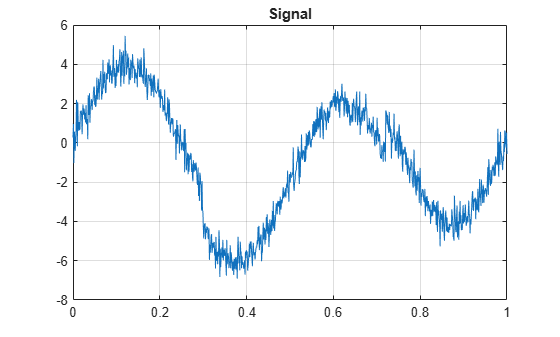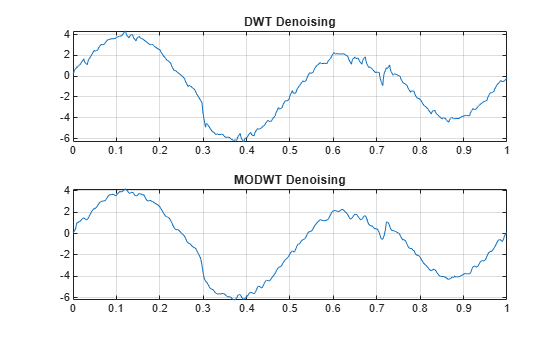# wden

Automatic 1-D denoising

`wden` is no longer recommended. Use `wdenoise` instead.

## Syntax

``XD = wden(X,TPTR,SORH,SCAL,N,wname)``
``XD = wden(C,L,___)``
``XD = wden(W,'modwtsqtwolog',SORH,'mln',N,wname)``
``[XD,CXD] = wden(___)``
``[XD,CXD,LXD] = wden(___)``
``[XD,CXD,LXD,THR] = wden(___)``
``[XD,CXD,THR] = wden(___)``

## Description

example

````XD = wden(X,TPTR,SORH,SCAL,N,wname)` returns a denoised version `XD` of the signal `X`. The function uses an `N`-level wavelet decomposition of `X` using the specified orthogonal or biorthogonal wavelet `wname` to obtain the wavelet coefficients. The thresholding selection rule `TPTR` is applied to the wavelet decomposition. `SORH` and `SCAL` define how the rule is applied.```
````XD = wden(C,L,___)` returns a denoised version `XD` of the signal `X` using the same options as in the previous syntax, but obtained directly from the wavelet decomposition structure [`C`,`L`] of `X`. [`C`,`L`] is the output of `wavedec`.```
````XD = wden(W,'modwtsqtwolog',SORH,'mln',N,wname)` returns the denoised signal `XD` obtained by operating on the maximal overlap discrete wavelet transform (MODWT) matrix `W`, where `W` is the output of `modwt`. You must use the same orthogonal wavelet in both `modwt` and `wden`.```
````[XD,CXD] = wden(___)` returns the denoised wavelet coefficients. For discrete wavelet transform (DWT) denoising, `CXD` is a vector (see `wavedec`). For MODWT denoising, `CXD` is a matrix with `N`+1 rows (see `modwt`). The number of columns of `CXD` is equal to the length of the input signal `X`.```
````[XD,CXD,LXD] = wden(___)` returns the number of coefficients by level for DWT denoising. See `wavedec` for details. The `LXD` output is not supported for MODWT denoising. The additional output arguments `[CXD,LXD]` are the wavelet decomposition structure (see `wavedec` for more information) of the denoised signal `XD`.```
````[XD,CXD,LXD,THR] = wden(___)` returns the denoising thresholds by level for DWT denoising.```
````[XD,CXD,THR] = wden(___)` returns the denoising thresholds by level for MODWT denoising when you specify the `'modwtsqtwolog'` input argument.```

## Examples

collapse all

This example shows how to apply three different denoising techniques to a noisy signal. It compares the results with plots and the threshold values produced by each technique.

First, to ensure reproducibility of results, set a seed that will be used to generate the random noise.

`rng('default')`

Create a signal consisting of a 2 Hz sine wave with transients at 0.3 and 0.72 seconds. Add randomly generated noise to the signal and plot the result.

```N = 1000; t = linspace(0,1,N); x = 4*sin(4*pi*t); x = x - sign(t-0.3) - sign(0.72-t); sig = x + 0.5*randn(size(t)); plot(t,sig) title('Signal') grid on```Using the `sym8` wavelet, perform a level 5 wavelet decomposition of the signal and denoise it by applying three different threshold selection rules to the wavelet coefficients: SURE, minimax, and Donoho and Johnstone's universal threshold with level-dependent estimation of the noise. In each case, apply hard thresholding.

```lev = 5; wname = 'sym8'; [dnsig1,c1,l1,threshold_SURE] = wden(sig,'rigrsure','h','mln',lev,wname); [dnsig2,c2,l2,threshold_Minimax] = wden(sig,'minimaxi','h','mln',lev,wname); [dnsig3,c3,l3,threshold_DJ] = wden(sig,'sqtwolog','h','mln',lev,wname);```

Plot and compare the three denoised signals.

```subplot(3,1,1) plot(t,dnsig1) title('Denoised Signal - SURE') grid on subplot(3,1,2) plot(t,dnsig2) title('Denoised Signal - Minimax') grid on subplot(3,1,3) plot(t,dnsig3) title('Denoised Signal - Donoho-Johnstone') grid on```Compare the thresholds applied at each detail level for the three denoising methods.

`threshold_SURE`
```threshold_SURE = 1×5 0.9592 0.6114 1.4734 0.7628 0.4360 ```
`threshold_Minimax`
```threshold_Minimax = 1×5 1.1047 1.0375 1.3229 1.1245 1.0483 ```
`threshold_DJ`
```threshold_DJ = 1×5 1.8466 1.7344 2.2114 1.8798 1.7524 ```

This example denoises a signal using the DWT and MODWT. It compares the results with plots and the threshold values produced by each technique.

First, to ensure reproducibility of results, set a seed that will be used to generate random noise.

`rng('default')`

Create a signal consisting of a 2 Hz sine wave with transients at 0.3 and 0.72 seconds. Add randomly generated noise to the signal and plot the result.

```N = 1000; t = linspace(0,1,N); x = 4*sin(4*pi*t); x = x - sign(t-0.3) - sign(0.72-t); sig = x + 0.5*randn(size(t)); plot(t,sig) title('Signal') grid on```Using the `db2` wavelet, perform a level 3 wavelet decomposition of the signal and denoise it using Donoho and Johnstone's universal threshold with level-dependent estimation of the noise. Obtain denoised versions using DWT and MODWT, both with soft thresholding.

```wname = 'db2'; lev = 3; [xdDWT,c1,l1,threshold_DWT] = wden(sig,'sqtwolog','s','mln',lev,wname); [xdMODWT,c2,threshold_MODWT] = wden(sig,'modwtsqtwolog','s','mln',lev,wname);```

Plot and compare the results.

```subplot(2,1,1) plot(t,xdDWT) grid on title('DWT Denoising') subplot(2,1,2) plot(t,xdMODWT) grid on title('MODWT Denoising')```Compare the thresholds applied in each case.

`threshold_DWT`
```threshold_DWT = 1×3 1.7783 1.6876 2.0434 ```
`threshold_MODWT`
```threshold_MODWT = 1×3 1.2760 0.6405 0.3787 ```

This example denoises a blocky signal using the Haar wavelet with DWT and MODWT denoising. It compares the results with plots and metrics for the original and denoised versions.

First, to ensure reproducibility of results, set a seed that will be used to generate random noise.

`rng('default')`

Generate a signal and a noisy version with the square root of the signal-to-noise ratio equal to 3. Plot and compare each.

```[osig,nsig] = wnoise('blocks',10,3); plot(nsig,'r') hold on plot(osig,'b') legend('Noisy Signal','Original Signal')```Using the Haar wavelet, perform a level 6 wavelet decomposition of the noisy signal and denoise it using Donoho and Johnstone's universal threshold with level-dependent estimation of the noise. Obtain denoised versions using DWT and MODWT, both with soft thresholding.

```wname = 'haar'; lev = 6 ; [xdDWT,c1,l1] = wden(nsig,'sqtwolog','s','mln',lev,wname); [xdMODWT,c2] = wden(nsig,'modwtsqtwolog','s','mln',lev,wname);```

Plot and compare the original, noise-free version of the signal with the two denoised versions.

```figure plot(osig,'b') hold on plot(xdDWT,'r--') plot(xdMODWT,'k-.') legend('Original','DWT','MODWT') hold off```Calculate the L2 and L-infinity norms of the difference between the original signal and the two denoised versions.

`L2norm_original_DWT = norm(abs(osig-xdDWT),2)`
```L2norm_original_DWT = 36.1194 ```
`L2norm_original_MODWT = norm(abs(osig-xdMODWT),2)`
```L2norm_original_MODWT = 14.5987 ```
`LInfinity_original_DWT = norm(abs(osig-xdDWT),Inf)`
```LInfinity_original_DWT = 4.7181 ```
`LInfinity_original_MODWT = norm(abs(osig-xdMODWT),Inf)`
```LInfinity_original_MODWT = 2.9655 ```

## Input Arguments

collapse all

Input data to denoise, specified as a real-valued vector.

Data Types: `double`

Wavelet expansion coefficients of the data to be denoised, specified as a real-valued vector. `C` is the output of `wavedec`.

Example: `[C,L] = wavedec(randn(1,1024),3,'db4')`

Data Types: `double`

Size of wavelet expansion coefficients of the signal to be denoised, specified as a vector of positive integers. `L` is the output of `wavedec`.

Example: `[C,L] = wavedec(randn(1,1024),3,'db4')`

Data Types: `double`

Maximal overlap wavelet decomposition structure of the signal to denoise, specified as a real-valued matrix. `W` is the output of `modwt`. You must use the same orthogonal wavelet in both `modwt` and `wden`.

Data Types: `double`

Threshold selection rule to apply to the wavelet decomposition structure of `X`:

• `'rigsure'` — Use the principle of Stein's Unbiased Risk.

• `'heursure'` — Use a heuristic variant of Stein's Unbiased Risk.

• `'sqtwolog` — Use the universal threshold $\sqrt{2\mathrm{ln}\left(\text{length}\left(x\right)\right)}.$

• `'minimaxi'` — Use minimax thresholding. (See `thselect` for more information.)

Type of thresholding to perform:

• `'s'` — Soft thresholding

• `'h'` — Hard thresholding

Multiplicative threshold rescaling:

• `'one'` — No rescaling

• `'sln'` — Rescaling using a single estimation of level noise based on first-level coefficients

• `'mln'` — Rescaling using a level-dependent estimation of level noise

Level of wavelet decomposition, specified as a positive integer. Use `wmaxlev` to ensure that the wavelet coefficients are free from boundary effects. If boundary effects are not a concern in your application, a good rule is to set `N` less than or equal to `fix(log2(length(X)))`.

Name of wavelet, specified as a character array, to use for denoising. For DWT denoising, the wavelet must be orthogonal or biorthogonal. For MODWT denoising, the wavelet must be orthogonal. Orthogonal and biorthogonal wavelets are designated as type 1 and type 2 wavelets, respectively, in the wavelet manager, `wavemngr`.

• Valid built-in orthogonal wavelet families are: Best-localized Daubechies (`"bl"`), Beylkin (`"beyl"`), Coiflets (`"coif"`), Daubechies (`"db"`), Fejér-Korovkin (`"fk"`), Haar (`"haar"`), Han linear-phase moments (`"han"`), Morris minimum-bandwidth (`"mb"`), Symlets (`"sym"`), and Vaidyanathan (`"vaid"`).

• Valid built-in biorthogonal wavelet families are: Biorthogonal Spline (`"bior"`), and Reverse Biorthogonal Spline (`"rbio"`).

For a list of wavelets in each family, see `wfilters`. You can also use `waveinfo` with the wavelet family short name. For example, `waveinfo("db")`. Use `wavemngr("type",wn)` to determine if the wavelet wn is orthogonal (returns 1) or biorthogonal (returns 2). For example, `wavemngr("type","db6")` returns 1.

## Output Arguments

collapse all

Denoised data, returned as a real-valued vector.

Data Types: `double`

Denoised wavelet coefficients, returned as a real-valued vector or matrix. For DWT denoising, `CXD` is a vector (see `wavedec`). For MODWT denoising, `CXD` is a matrix with `N+1` rows (see `modwt`). The number of columns is equal to the length of the input signal `X`.

Data Types: `double`

Size of denoised wavelet coefficients by level for DWT denoising, returned as a vector of positive integers (see `wavedec`). The `LXD` output is not supported for MODWT denoising. `[CXD,LXD]` is the wavelet decomposition structure of the denoised signal `XD`.

Data Types: `double`

Denoising thresholds by level, returned as a length `N` real-valued vector.

Data Types: `double`

## Algorithms

The most general model for the noisy signal has the following form:

`$s\left(n\right)=f\left(n\right)+\sigma e\left(n\right),$`

where time n is equally spaced. In the simplest model, suppose that e(n) is a Gaussian white noise N(0,1), and the noise level σ is equal to 1. The denoising objective is to suppress the noise part of the signal s and to recover f.

The denoising procedure has three steps:

1. Decomposition — Choose a wavelet, and choose a level `N`. Compute the wavelet decomposition of the signal s at level `N`.

2. Detail coefficients thresholding — For each level from 1 to `N`, select a threshold and apply soft thresholding to the detail coefficients.

3. Reconstruction — Compute wavelet reconstruction based on the original approximation coefficients of level `N` and the modified detail coefficients of levels from 1 to `N`.

More details about threshold selection rules are in Wavelet Denoising and Nonparametric Function Estimation and in the help of the `thselect` function. Note that:

• The detail coefficients vector is the superposition of the coefficients of f and the coefficients of e. The decomposition of e leads to detail coefficients that are standard Gaussian white noises.

• Minimax and SURE threshold selection rules are more conservative and more convenient when small details of function f lie in the noise range. The two other rules remove the noise more efficiently. The option `'heursure'` is a compromise.

In practice, the basic model cannot be used directly. To deal with model deviations, the remaining parameter `scal` must be specified. It corresponds to threshold rescaling methods.

• The option `scal` = `'one'` corresponds to the basic model.

• The option `scal = 'sln'` handles threshold rescaling using a single estimation of level noise based on the first-level coefficients.

In general, you can ignore the noise level that must be estimated. The detail coefficients CD1 (the finest scale) are essentially noise coefficients with standard deviation equal to σ. The median absolute deviation of the coefficients is a robust estimate of σ. The use of a robust estimate is crucial. If level 1 coefficients contain f details, these details are concentrated in a few coefficients to avoid signal end effects, which are pure artifacts due to computations on the edges.

• The option `scal` = `'mln'` handles threshold rescaling using a level-dependent estimation of the level noise.

When you suspect a nonwhite noise e, thresholds must be rescaled by a level-dependent estimation of the level noise. The same kind of strategy is used by estimating σlev level by level. This estimation is implemented in the file `wnoisest`, which handles the wavelet decomposition structure of the original signal s directly.

 Antoniadis, A., and G. Oppenheim, eds. Wavelets and Statistics, 103. Lecture Notes in Statistics. New York: Springer Verlag, 1995.

 Donoho, D. L. “Progress in Wavelet Analysis and WVD: A Ten Minute Tour.” Progress in Wavelet Analysis and Applications (Y. Meyer, and S. Roques, eds.). Gif-sur-Yvette: Editions Frontières, 1993.

 Donoho, D. L., and Johnstone, I. M. “Ideal Spatial Adaptation by Wavelet Shrinkage.” Biometrika, Vol. 81, pp. 425–455, 1994.

 Donoho, D. L. “De-noising by Soft-Thresholding.” IEEE Transactions on Information Theory, Vol. 42, Number 3, pp. 613–627, 1995.

 Donoho, D. L., I. M. Johnstone, G. Kerkyacharian, and D. Picard. “Wavelet Shrinkage: Asymptopia?” Journal of the Royal Statistical Society, series B. Vol. 57, Number 2, pp. 301–369, 1995.